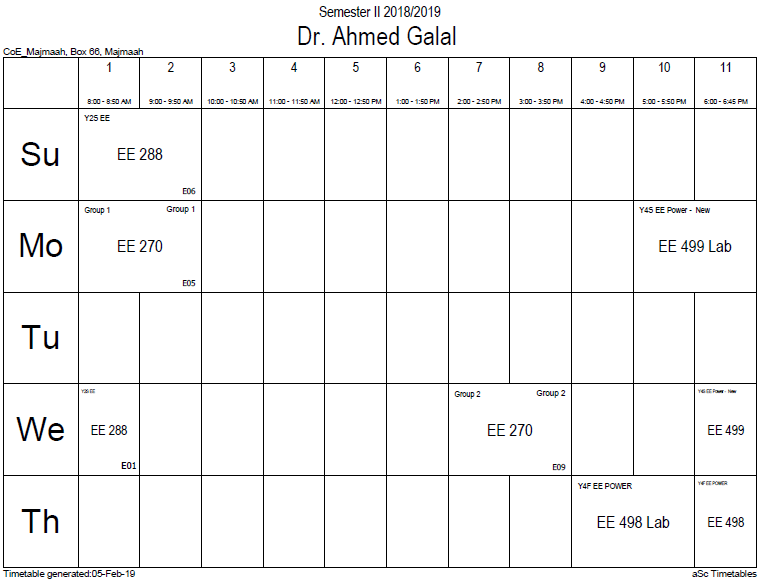## Magn comp. relucta

Magnetic complex reluctance (SI Unit: H−1) is a measurement of a passive magnetic circuit (or element within that circuit) dependent on sinusoidal magnetomotive force (SI Unit: At·Wb−1) and sinusoidal magnetic flux (SI Unit: T·m2), and this is determined by deriving the ratio of their complex effective amplitudes.[Ref. 1-3]$Z_mu = frac{dot N}{dot Phi} = frac{dot {N}_m}{dot {Phi}_m} = z_mu e^{jphi}$

As seen above, magnetic complex reluctance is a phasor represented as uppercase Z mu where:$dot N$ and$dot {N}_m$ represent the magnetomotive force (complex effective amplitude)$dot Phi$ and$dot {Phi}_m$ represent the magnetic flux (complex effective amplitude)$z_mu$, lowercase z mu, is the real part of magnetic complex reluctance

The "lossless" magnetic reluctance, lowercase z mu, is equal to the absolute value (modulus) of the magnetic complex reluctance. The argument distinguishing the "lossy" magnetic complex reluctance from the "lossless" magnetic reluctance is equal to the natural number$e$ raised to a power equal to:$jphi = jleft(eta - alpha ight)$

Where:

•$j$ is the imaginary number
•$eta$ is the phase of the magnetomotive force
•$alpha$ is the phase of the magnetic flux
•$phi$ is the phase difference

The "lossy" magnetic complex reluctance represents a magnetic circuit element's resistance to not only magnetic flux but also to changes in magnetic flux. When applied to harmonic regimes, this formality is similar to Ohm's Law in ideal AC circuits. In magnetic circuits, magnetic complex reluctance is equal to:$Z_mu = frac{1}{dot {mu} mu_0}frac{l}{S}$

Where:

•$l$ is the length of the circuit element
•$S$ is the cross-section of the circuit element
•$dot {mu} mu_0$ is the complex magnetic permeability

### Office HoursNo office hours### My Timetable### Contactsemail: [email protected]

Phone: 2570

### Welcome

Welcome To Faculty of Engineering### IEEEhttp://www.ieee.org/

/

### Bookmarkshttp://www.utk.edu/research/

http://science.doe.gov/grants/index.asp

http://www1.eere.energy.gov/vehiclesandfuels/

http://www.eere.energy.gov/

### Upcoming Conferences### Engineering quotes### Travel Web Siteshttp://www.hotels.com/

http://www.orbitz.com/

http://www.hotwire.com/us/index.jsp

http://www.kayak.com/

### Blackboardستقام اختبارات الميدتيرم يوم الثلاثاء 26-6-1440

حسب الجدول المعلن بلوحات الاعلان

### Summer trainingThe registration for summer training will start from 5th week of second semesterClass registration week 1

### برنامج التجسير### إحصائية الموقع

عدد الصفحات: 2879

البحوث والمحاضرات: 1280

الزيارات: 99616